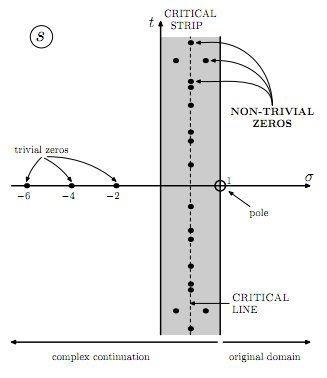### Riemann hypothesis

The Rieman hypethesis was stated following the 1859 Riemann's paper On the Number of Primes Less Than a Given Magnitude. This is the begin of the paper(1):
I believe that I can best convey my thanks for the honour which the Academy has to some degree conferred on me, through my admission as one of its correspondents, if I speedily make use of the permission thereby received to communicate an investigation into the accumulation of the prime numbers; a topic which perhaps seems not wholly unworthy of such a communication, given the interest which Gauss and Dirichlet have themselves shown in it over a lengthy period.
For this investigation my point of departure is provided by the observation of Euler that the product $\prod \frac{1}{1-\frac{1}{p^s}} = \sum \frac{1}{n^s}$ if one substitutes for $p$ all prime numbers, and for $n$ all whole numbers. The function of the complex variable $s$ which is represented by these two expressions, wherever they converge, I denote by $\zeta (s)$. Both expressions converge only when the real part of $s$ is greater than 1; at the same time an expression for the function can easily be found which always remains valid.The Riemann zeta function is connected to the prime numbers distribution, in particular Riemann argued that all of its non trivial zeros(2) have the form $z = \frac{1}{2} + bi$, where $z$ is complex, $b$real,$i = \sqrt{-1}$. There's also a general form of the zeros: $z = \sigma + bi$, where $\sigma$ belong to the critical strip (see below and the image at the right).
In the story of the search of the zeta-zeros, Hugh Montgomery has an important part(4): in 1972 he investigated the distance between two zeta-zeros, finding a function of this difference. After this paper, in 1979, with Norman Levinson(5) he established some others zeta properties, investigating in particular the zeros of zeta derivatives. Obviosly he first of all proofed an equivalence relation between the zeros of Riemann zeta function and the zeros of the derivatives: in particular also these zeros belong to the critical strip, $0 < \sigma < \frac{1}{2}$.
The analitical research around zeta-zeros is not the only way: the first was Lehmer (1956 and 1957) who performed the first computational attempt in order to proof the hypothesis. An example of this kind of researches is given by Richard Brent(6): in his work he try to evaluate Riemann zeta using the Gram points, that are the points in which the zeta change its sign(3). Brent focused his research on the first 70000000 Gram blocks, veryfing the hypothesis.
But there's another approach to the problem: physics. In the end of 90s Alain Connes(7) proofed the link of Rieman hypotesis with quantum chaos.
Quantum chaos studies chaotic classical dynamical systems using quantum laws. In particular Connes found a particular chaotic system in which quantum numbers are prime numbers and the energy levels of the system correspond to the zeta-zeros on the critical line $\sigma = \frac{1}{2}$. In physics it could be the better (but not only) suspect to resolve the hypothesis
Others connection with physics are in the review Physics of the Riemann Hypothesis by Daniel Schumayer and David A. W. Hutchinson, but we can speak about the stories in the paper in another moment.
(1) Translated by David R. Wilkins (pdf)
(2) In this case, for trivial zero I intend a negative even integer number.
(3) We can also define a Gram point as the value of $b$ such that $\zeta \left ( \frac{1}{2} + bi \right ) = Z(b) e^{-i \theta (b)}$ is a non-zero real.
In the equation $\theta(t) \sim \frac{t}{2}\log \frac{t}{2\pi} - \frac{t}{2} - \frac{\pi}{8}+\frac{1}{48t}+ \frac{7}{5760t^3}+\cdots$ is the Riemann-Siegel theta function and $Z (t)$is the Hardy Z-function.
(4) Montgomery, H. L. The pair correlation of zeros of the zeta function. Analytic number theory (Proc. Sympos. Pure Math., Vol. XXIV, St. Louis Univ., St. Louis, Mo., 1972), pp. 181–193. Amer. Math. Soc., Providence, R.I., 1973.
(5) Levinson, N., & Montgomery, H. (1974). Zeros of the derivatives of the Riemann zeta-function Acta Mathematica, 133 (1), 49-65 DOI: 10.1007/BF02392141
(6) Brent, R. (1979). On the zeros of the Riemann zeta function in the critical strip Mathematics of Computation, 33 (148), 1361-1361 DOI: 10.1090/S0025-5718-1979-0537983-2
(7) Connes, A. (1999). Trace formula in noncommutative geometry and the zeros of the Riemann zeta function Selecta Mathematica, 5 (1), 29-106 DOI: 10.1007/s000290050042 (arXiv)